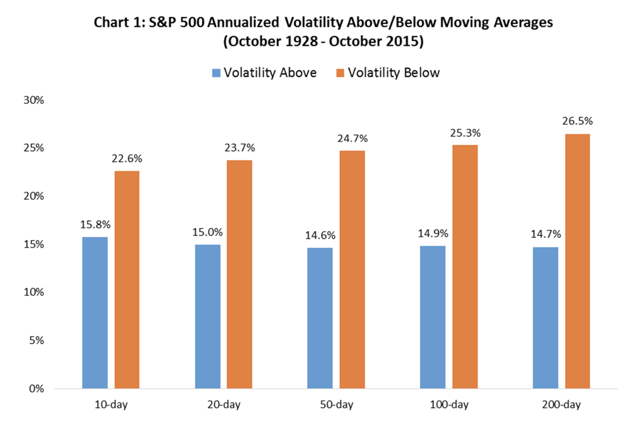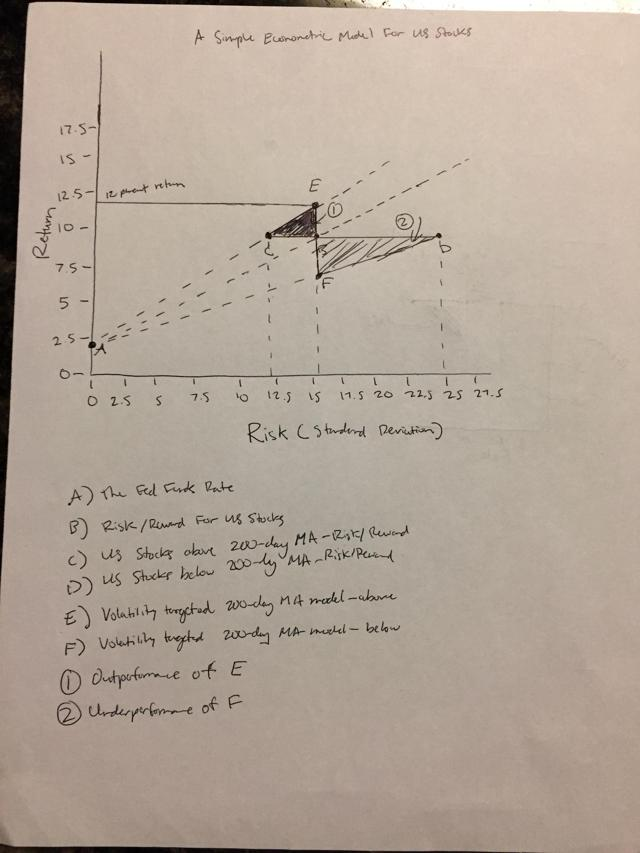Card Counting Meets The Stock Market: A Statistical Model For Predicting 1-3 Month Returns

|
Includes:
by: Logan Kane
Summary

A simple 3-part model may forecast stock returns.

The inputs are the risk-free rate, stock valuations, and a volatility forecast.

The output is how much risk to take over the next 1-3 months.

Anyone can apply this model to improve the risk-reward of their portfolio.

In this article, I'll share a simple model to forecast the returns and risk of the S&P 500. Using a good statistical model for stocks is not a crystal ball, but it should strongly outperform random chance.

1. Return Forecast

Stock returns are notoriously hard to forecast. Still, we can infer some things based on the state of the world at the moment. If you use valuation, for example, typically you can explain about 15-20 percent of the next quarter's returns from the forward price to earnings ratio of the market. This actually isn't as bad as it sounds because it's better than guessing, but we can only weakly forecast where stocks will be in the future. Long run expected returns aren't as hard to forecast as you think. For expected returns, I simply use the earnings yield of the S&P 500 plus a conservative expectation for earnings growth. This is roughly in-line with historical averages, and it works pretty well when I test it on random time periods, too. This fluctuates a little bit month by month, but last time I calculated it, it was 9.5 percent.

However, if you combine a weak return forecast with stronger forecasts for interest rates and volatility over a 1-3 month timeframe, you get a much stronger forecast. You can use this historically to beat the market by 3-5 percent per year for the same level of risk.

2. Volatility Forecast

Volatility is surprisingly easy to forecast due to the strong auto-correlation (today's volatility=tomorrow's volatility). If this month is volatile, next month is likely to be somewhat volatile as well. Volatility also tends to rise when stocks have fallen over the previous few months.

One simple way to forecast the volatility of the market is to use the 200-day moving average as a barometer. For the volatility forecast, I use an educated guess for volatility when the S&P is above its moving average and an educated guess for volatility when the index is below based on historical data.Source: Pension Partners

As you can see, volatility is historically much higher when stocks are falling. The trick to making money from this is to treat volatility the way card counters treat the count of the blackjack deck. When volatility is low, you take more risk, by allocating more to stocks in a stock/bond portfolio, by using leveraged ETFs, or my personal favorite, E-mini S&P 500 futures. When volatility is high (like 2001 and 2008), you take less risk until things settle down. The current environment is fairly conducive to risk, as volatility has only averaged 12-13 percent since 2010. Central banks are more willing now than they have been in the past to intervene when things get rough for stocks. I view this as a positive. In any case, volatility is currently somewhat suppressed.

3. Interest Rate Forecast

The other variable you need to be aware of is the risk-free rate of interest. The current/expected fed funds rate is priced into futures and options, so we can get a good idea of where it will be over the next few months if using ETFs, or a perfect view if we're using futures because it's what is priced into the contracts. Any time you put money in stocks, you're foregoing the interest you would earn by keeping it in cash. If you use leverage, the risk-free rate of interest is priced into your cost of borrowing, so you pay it out of pocket. Either way, the current risk-free rate is subtracted from your returns. As such, professional traders like to look at the returns of strategies in excess of cash. For the interest rate forecast, I would use either the 3-month Treasury bill rate or the LIBOR.

4. Building The Model

I took the liberty of building a simple model based on expected returns, volatility, and the risk-free rate of interest. It's likely that someone before me has come up with a similar model, but I like the way I did it for simplicity and elegance. I sketched out the current (June 2019) forecast by hand below.

Here's the key to the points on my graph below.

A- The risk-free rate of interest.

B- The expected long-term risk and return of the S&P 500.

C- The expected risk and return of the S&P 500 when the index is above its 200-day moving average.

D- The expected risk and return of the S&P 500 when the index is below its 200-day moving average.

E- The return and risk if you use low-risk environments to take more equity risk.

F- The return and risk if you did the opposite and scaled the strategy to the same volatility target, buying stocks only when they fall and selling when things turn around.

1) The upper triangle is the amount of alpha from timing the market.

2) The second triangle below, on the right is the size of underperformance from running the opposite strategy. The triangles are directly related but different in size because markets spend about 2/3rds of the time above their 200-day moving average.

The slope of the line between A and points E, B and D are the Sharpe ratios of each strategy. You can see quite clearly that as interest rates rise, stocks become less attractive. The model responds by showing less benefit for leverage after each interest rate hike, or if the cash rate is above the expected rate of return on stocks (like in 2000 or the early 1980s, you're out of stocks and move to cash/bonds until rates come down). You can readily compare this with the expected Sharpe ratio of bonds and other trading strategies to know when equity risk is most likely to most rewarded. Below is the current forecast. It's good for the next month. Note, however, that the model no longer fits when you get above roughly 20 percent monthly annualized volatility, due to an effect called volatility drag.

As such, you can currently beat the market by about 3 percent per year over the long-run (12 to 12.5 percent annual projected return vs. 9.5 percent annual projected return) by avoiding owning stocks when they are below their 200-day moving average.5. Implementation

The cleanest implementation is to use futures to dial in the exact amount of risk you want to take and to be able to borrow close to the risk-free rate. When stocks are above the moving average, you own them and target volatility. When stocks are below, you own Treasuries and target a duration that would result in similar volatility. This actually doubles the outperformance to about 6 percent per year because the Fed cuts rates more often when the market is in a downtrend, playing into the hands of Treasury holders.

The easiest implementation for most people reading this is to use a combination of (SPY) and (UPRO) when the S&P is above the 200-day moving average and to invest in Treasuries via the ETFs (IEF) or (TLT) when the index is below its 200-day moving average. You can set whatever leverage level and volatility target you're comfortable with.

To reduce noise, I would suggest you consider using some kind of moving average crossover to trigger trades. Let me know if you guys like this article–if you do I may share my model for US Treasuries also in the future.